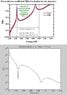# Matlab Plotting of QM double-potential-barrier

• MATLAB
• dLo R6

#### dLo R6

Hey everyone, I have a quantum mechanics/matlab issue. I'm trying to plot the Transmission Coefficient T(E) of a double-potential barrier with barrier width 3nm and spacing 9nm, potential V = 0.5eV and sweeping energy from 0 to 1eV. Using the cascading matrix method where, given a matrix M of each interface, the beginning and end coefficients of the wavefunctions can be found, with the end wavefunction having a coefficient t and |t|^2 gives the transmission. One would have to probably be familiar with this QM problem to get the whole idea.

Anyway, I wrote out a function to do just what was described, and came across an oddity. I get the correct graph, except it's flipped upside down and rather than being between 0 and 1, it's between infinity and 1, but on the correct scale. see the image below; can anyone pick through this code and possibly see where I've gone wrong or why this graph is showing up the way it is? if someone had some free time i'd really appreciate it, I've been hitting my head against the wall for 2 days now. thanks!

attached image
top: desired plot
bottom: my plot (notice the y axis)

code
%EE270 HW3 Problem 1
% Ryan Dumlao
%Consider a double barrier quantum well with a well width of L= 9 nm,
%barrier widths of d=3 nm, and a barrier height of V0=500 meV. Assume
%that you are considering the GaAs/AlGaAs material system, with an
%electronic effective mass of m*=0.067m0.
clc;

%code for getting a(n) vector
syms x1 x2 x3 x4 x5 aip ain Vo Eo L d r;
N = 2; %number of barriers
x = zeros(2*N);
%for 2-barrier only
L = 10e-9;
d = 10e-9;
x = [-(L/2+d) -L/2 L/2 L/2+d]';
%x = [x1 x2 x3 x4 x5];
Mi = zeros(2,2);
Mf = eye(2,2);
hbar = 6.626e-34/(2*pi);
me = 9.109e-31;
m = 0.067*me;
syms k1 k2 k3 k4 k5;
k = zeros(2*N+1,1);
V = zeros(2*N+1,1);
ai = zeros(2,1);
af = zeros(2,1);
ai = [1;.28];

%funsies
%E1e = hbar^2*pi*2/(2*m*d^2)*6.241e18
%E1GaAs = hbar^2*pi*2/(2*me*d^2)*6.241e18

%fill the potential barrier potential energy vector
for n = 1:2:2*N+1,
V(n) = 0;
V(n+1) = 0.100*1.602e-19;
end

%loop for many energies
for p = 1:1:1400,
E = p*.0001*1.602e-19;
%for each loop we will calculate the M for going in then out of one
%barrier
k(1) = sqrt(2*m*(E-V(1))/hbar^2);
k(2) = sqrt(2*m*(E-V(2))/hbar^2);
k(3) = k(1);
kmax(p) = k(2);
kmin(p) = k(1);

% pass into barrier 1
A = [exp(i*k(1)*x(1)) exp(-i*k(1)*x(1));
i*k(1)*exp(i*k(1)*x(1)) -i*k(1)*exp(-i*k(1)*x(1))];
B = [exp(i*k(2)*x(1)) exp(-i*k(2)*x(1));
i*k(2)*exp(i*k(2)*x(1)) -i*k(2)*exp(-i*k(2)*x(1))];
M1 = inv(B)*A;

% pass out of barrier 1
C = [exp(i*k(2)*x(2)) exp(-i*k(2)*x(2));
i*k(2)*exp(i*k(2)*x(2)) -i*k(2)*exp(-i*k(2)*x(2))];
D = [exp(i*k(1)*x(2)) exp(-i*k(1)*x(2));
i*k(1)*exp(i*k(1)*x(2)) -i*k(1)*exp(-i*k(1)*x(2))];
M2 = inv(D)*C;

% pass into barrier 2
F = [exp(i*k(1)*x(3)) exp(-i*k(1)*x(3));
i*k(1)*exp(i*k(1)*x(3)) -i*k(1)*exp(-i*k(1)*x(3))];
G = [exp(i*k(2)*x(3)) exp(-i*k(2)*x(3));
i*k(2)*exp(i*k(2)*x(3)) -i*k(2)*exp(-i*k(2)*x(3))];
M3 = inv(G)*F;

% pass out of barrier 2
H = [exp(i*k(2)*x(4)) exp(-i*k(2)*x(4));
i*k(2)*exp(i*k(2)*x(4)) -i*k(2)*exp(-i*k(2)*x(4))];
J = [exp(i*k(1)*x(4)) exp(-i*k(1)*x(4));
i*k(1)*exp(i*k(1)*x(4)) -i*k(1)*exp(-i*k(1)*x(4))];
M4 = inv(J)*H;

Mtot = M4*M3*M2*M1;
af=Mtot*ai;
tt(p) = Mtot(1,1)-Mtot(2,1)/Mtot(2,2);
T2(p) = abs(tt(p))^2;
%T(p) = -abs(af(1))^2;
En(p) = p;
end

figure(1);
%semilogy(En/10000,T);
%hold;
semilogy(En,T2,'r');
title('T(E) Double Barrier, d = 3nm L = 9nm, V = 0.5 eV')
xlabel('Energy (eV)');
ylabel('T(E)');

#### Attachments

•results.JPG
26.3 KB · Views: 1,166
maybe you can help me with a program?
im trying to plot the transmittion coeffiecet vs energy
for an arbitrary seriers of step potencials with the same step potencial and length

please i have another problem i want to get the graph of energy vs barrier width .i hav done the program in wolferm methamatica but cannot find the output .please help